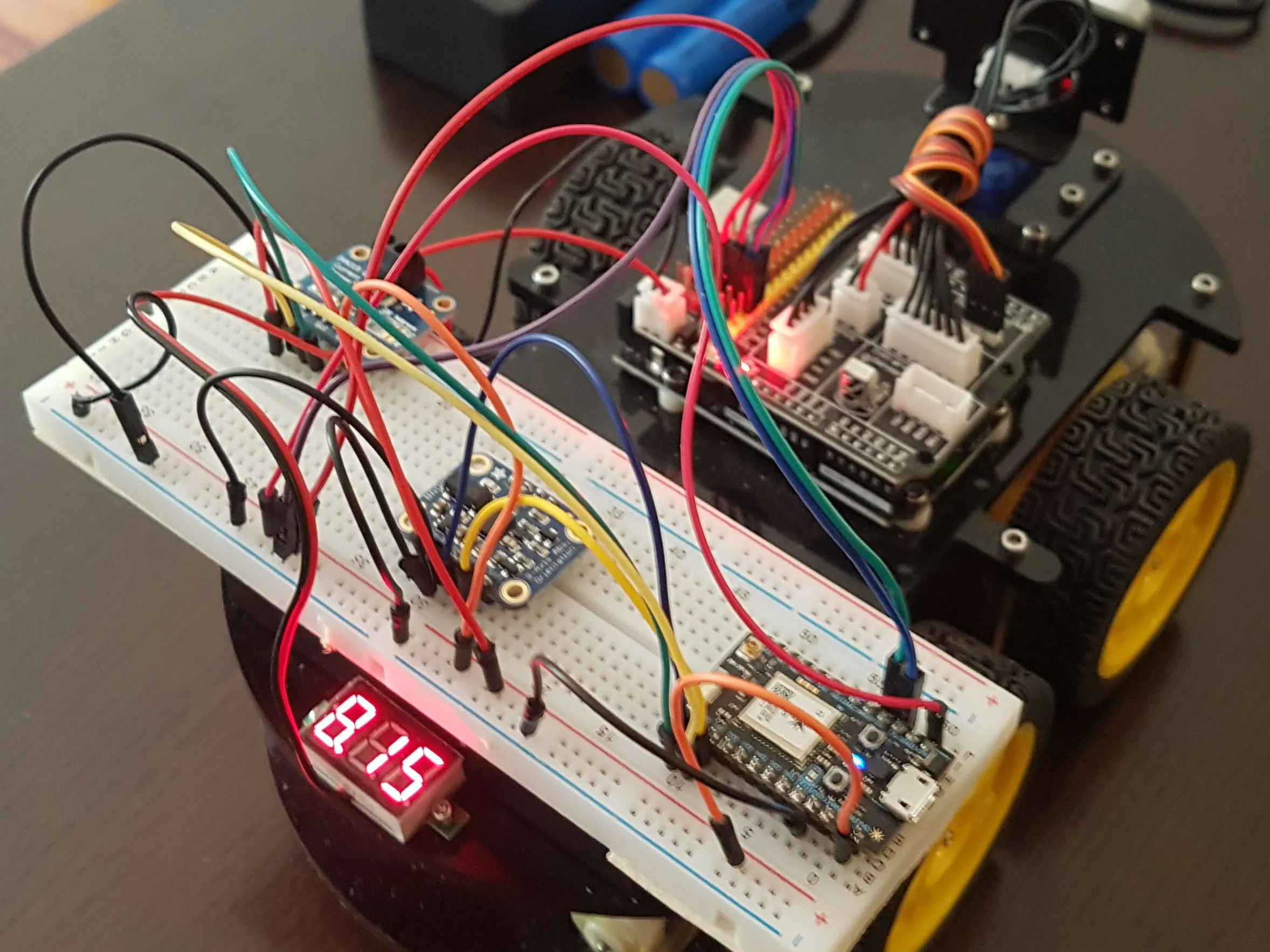# Robot Car Example with Arduino and Photon

In this project, I will share some of the techniques used by my low-cost robot car projects.## Things used in this project

### Hardware components

 Generic robot car kit Any of many Arduino-based robot car kits. Either two or four wheeled, does not make much difference. Things to look for are 18650 battery holder, motors and motor control circuitry.
×1Particle Photon
×1×1
 SparkFun Digital volt meter (optional)
×1

### Software apps and online services

 Particle Cloud

## Schematics

### Serial connection

Connecting an Arduino and a Core, Photon or Electron. In this Fritzing circuit file the Arduino acts as the power source for the Photon.

## Code

### Arduino code

Arduino
This is the code for the Arduino. It simply sends all data from the sensors connected to the Arduino to the Photon via Serial. Likewise it accepts commands from the Photon via Serial and sets the motor and servo values.
```#include <Servo.h>  //servo library
Servo echoServo; // create servo object

int Echo = A4;
int Trig = A5;

#define LT_R 10
#define LT_M 4
#define LT_L 2

#define ENA 5
#define ENB 6
#define IN1 7
#define IN2 8
#define IN3 9
#define IN4 11

int ang = 1500;
int dist;
int ltr, mtr, rtr;
int ena = 0, enb = 0, inx = 0;
int stopMil;
unsigned long lastUpdate;

void allStop() {
digitalWrite(ENA, ena = 0);
digitalWrite(ENB, enb = 0);
digitalWrite(IN1, LOW);
digitalWrite(IN2, LOW);
digitalWrite(IN3, LOW);
digitalWrite(IN4, LOW);
inx = 0x0000;
}

// Ultrasonic distance measurement function

int distanceTest() {
digitalWrite(Trig, LOW);
delayMicroseconds(2);
digitalWrite(Trig, HIGH);
delayMicroseconds(20);
digitalWrite(Trig, LOW);
float Fdistance = pulseIn(Echo, HIGH, 25000) / 58;
return (int) Fdistance;
}

void setup() {
echoServo.attach(3); // attach servo on pin 3 to servo object
echoServo.write(ang); //set servo position according to scaled value
pinMode(Echo, INPUT);
pinMode(Trig, OUTPUT);
pinMode(IN1, OUTPUT);
pinMode(IN2, OUTPUT);
pinMode(IN3, OUTPUT);
pinMode(IN4, OUTPUT);
pinMode(ENA, OUTPUT);
pinMode(ENB, OUTPUT);
pinMode(LT_R, INPUT);
pinMode(LT_M, INPUT);
pinMode(LT_L, INPUT);
allStop();
Serial.begin(115200);
}

void loop() {
if ((millis() - lastUpdate) > 50) {
lastUpdate = millis();
// my time
Serial.print("M");
Serial.println(lastUpdate);
// distance
dist = distanceTest();
Serial.print("d");
Serial.println(dist, DEC);
// line tracking
Serial.print("t");
Serial.print(ltr, DEC);
Serial.print(mtr, DEC);
Serial.println(rtr, DEC);
}
}

void serialEvent() {
while (Serial.available()) {
case 'A':
{
ena = Serial.parseInt();
ena = constrain(ena, 0, 255);
analogWrite(ENA, ena);
break;
}
case 'B':
{
enb = Serial.parseInt();
enb = constrain(enb, 0, 255);
analogWrite(ENB, enb);
break;
}
case 'I':
{
inx = Serial.parseInt();
inx &= 0x1111;
digitalWrite(IN1, !(inx & 0x1000));
digitalWrite(IN2, !(inx & 0x0100));
digitalWrite(IN3, !(inx & 0x0010));
digitalWrite(IN4, !(inx & 0x0001));
break;
}
case 'a':
{
ang = Serial.parseInt();
ang = constrain(ang, 500, 2500);
echoServo.writeMicroseconds(ang);
break;
}
case 'e':
{
// echo settings back to the controller
// motor values
Serial.print("A");
Serial.println(ena);
Serial.print("B");
Serial.println(enb);
Serial.print("I");
Serial.println(inx);
// echo servo position
Serial.print("a");
Serial.println(ang, DEC);
break;
}
case 's': {
stopMil = Serial.parseInt();
stopMil = constrain(stopMil, 0, 49);
if (stopMil > 0) {
delay(stopMil);
}
allStop();
break;
}
// default: trash, ignore
}
}
}
```

### Photon code

C/C++
This is the code for the Photon. This is working code for me but will need quite some tweaking before you can use it in your own project.

What happens here is that we collect data from the Arduino, which includes sensor data from the robot, and pass that on via WiFi and UDP to a computer. Likewise, we collect some data from sensors attached to the Photon directly and pass those on as well. Finally, incoming commands from the computer are forwarded to the Arduino.
```// This #include statement was automatically added by the Particle IDE.

/*
Connections
===========
Connect SCL to D1
Connect SDA to D0
Connect VDD to 3.3V DC
Connect GROUND to common ground
*/

/* Set the delay between fresh samples */
#define BNO055_SAMPLERATE_DELAY_MS 25
#define LED_PIN D7 // (Particle is D7)
#define MY_PORT 2221
#define SERVER_PORT 2222

byte server[] = {192, 168, 178, 33};
boolean ledState = false;
unsigned long lastTime = 0;
unsigned long lastSec = 0;
UDP Udp;
byte toBuf;
int toOfs = 0;
imu::Vector<3> accelV;
imu::Vector<3> magV;
imu::Vector<3> gyroV;
imu::Vector<3> eulerV;
imu::Vector<3> linV;
imu::Vector<3> graV;

void toggleLED() {
ledState = !ledState;
digitalWrite(LED_PIN, ledState);
}

/**************************************************************************/
/*
Arduino setup function (automatically called at startup)
*/

/**************************************************************************/
void setup(void) {
pinMode(LED_PIN, OUTPUT);
Serial1.begin(115200);
Serial1.write('s');
Serial1.write('0');
Serial1.write('\n');
ina219.begin();
/* Initialise the sensor */
while (!bno.begin()) {
/* There was a problem detecting the BNO055 ... check your connections */
Particle.publish("bno055", "Not yet connected to BNO055.");
delay(1500);
}
Udp.begin(MY_PORT);
delay(1000);
bno.setExtCrystalUse(true);
Particle.publish("bno055", "Main code loop started.");
lastTime = lastSec = millis();
}

/**************************************************************************/
/*
Arduino loop function, called once 'setup' is complete (your own code
should go here)
*/

/**************************************************************************/
void loop(void) {
// Possible vector values can be:
// - VECTOR_ACCELEROMETER - m/s^2
// - VECTOR_MAGNETOMETER  - uT
// - VECTOR_EULER         - degrees
// - VECTOR_LINEARACCEL   - m/s^2
// - VECTOR_GRAVITY       - m/s^2
se();
rep();
}

void rep() {
if (millis() - lastTime > BNO055_SAMPLERATE_DELAY_MS) {
lastTime = millis();
float shuntvoltage = ina219.getShuntVoltage_mV();
float busvoltage = ina219.getBusVoltage_V();
float current_mA = ina219.getCurrent_mA();
Udp.beginPacket(server, SERVER_PORT);
Udp.write(String::format("m%lu\n", millis()));
Udp.write(String::format("V%f %f %f\n", shuntvoltage, busvoltage, current_mA));
if (lastTime - lastSec > 1000) {
lastSec = lastTime;
uint8_t systm, gyro, accel, mag;
bno.getCalibration(&systm, &gyro, &accel, &mag);
Udp.write(String::format("C%d %d %d %d\n", systm, gyro, accel, mag));
Udp.write(String::format("T%d\n", bno.getTemp()));
Udp.write(String::format("F%lu\n", System.freeMemory()));
}
Udp.write(String::format("p%f %f %f\n", accelV.x(), accelV.y(), accelV.z()));
Udp.write(String::format("q%f %f %f\n", magV.x(), magV.y(), magV.z()));
Udp.write(String::format("g%f %f %f\n", gyroV.x(), gyroV.y(), gyroV.z()));
Udp.write(String::format("G%f %f %f\n", eulerV.x(), eulerV.y(), eulerV.z()));
Udp.write(String::format("L%f %f %f\n", linV.x(), linV.y(), linV.z()));
Udp.write(String::format("l%f %f %f\n", graV.x(), graV.y(), graV.z()));
Udp.endPacket();
}
}

void se() {
while (Serial1.available()) {
if (Serial1.available()) {
toBuf[toOfs++] = b;
if (b == 10) {
Udp.sendPacket(toBuf, toOfs, server, SERVER_PORT);
toOfs = 0;
}
}
}
int rx = Udp.parsePacket();
while (rx > 0) {